# Arithmetic - math word problems

1. On the tree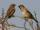15 squids and sparrows sat on the tree. Sparrows were 8 less than squids. How many sparrows were sitting at the tree?
2. Garden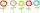The garden grows 312 roses and 389 tulips. Which flowers are more? How many?
3. Book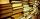Jitka read on holidays book that has 180 pages. In the first week read 45 pages. In the second week she read 15 pages more than the first week. How many pages left to read it yet?
4. Series and sequencesFind a fraction equivalent to the recurring decimal? 0.435643564356
5. Parabola 3Find the equation of a parabola with its focus at (0,2) and its vertex at the origin. ?
6. Thermometerthermometer showed -12 degrees Celsius at the morning then the temperature rises by 4 degrees and later again increased by 2 degrees at the evening has fallen by 5 degrees and then fall 3 degrees. What end temperature does the thermometer show?
7. Money split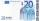Anton and Ferko got 2,500 euros together. The amount was split in 1:4 ratio. How much did each get?
8. MO Z8–I–6 2018In the KLMN trapeze, KL has a 40 cm base and an MN of 16 cm. Point P lies on the KL line so that the NP segment divides the trapezoid into two parts with the same area. Find the length of the KP line.
9. Shirt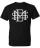Mrs. Vítková bought each of his three children the same shirt paid CZK 1,000. Saleswoman she returned 568,60 CZK. What was the price of one shirt?
10. QuartersHow many meters is a quarter of a kilometer? How many centimeters is a quarter of a meter, how many cents is a quarter of the euro?
11. Big number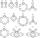hat is the remainder when dividing number 10 to 47 - 111 by number 9?
12. Solve equationsolve equation: ?
13. Dividing by five and tenNumber 5040 divide by the number 5 and by number 10: a = 5040: 5 b = 5040: 10
14. DiagonalsA diagonal of a rhombus is 20 cm long. If it's one side is 26 cm find the length of the other diagonal.
15. Points on line segmentPoints P & Q belong to segment AB. If AB=a, AP = 2PQ = 2QB, find the distance: between point A and the midpoint of the segment QB.
16. Unknown number 17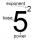Milada said, I am thinking of a number such that I evaluate expression x1/3, the value of the expression would be 5. Which number Milada thinking?
17. FlowersThe flower has six flowers and each flower has a number. These are the numbers: 20,40,39,28,8,9. What number will be in the middle of the flower so that the numbers come from the flowers when we subtract and add?
18. Product of the sum and differenceCalculate the product of the sum and difference of numbers -7 and -2.
19. MultiplesFind all multiples of 10 that are larger than 136 and smaller than 214.
20. Pie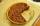Vili ate three pieces of pie . If it piece is 1/8 how much pie did he eat?

Do you have an interesting mathematical word problem that you can't solve it? Enter it, and we can try to solve it.

To this e-mail address, we will reply solution; solved examples are also published here. Please enter the e-mail correctly and check whether you don't have a full mailbox.

Please do not submit problems from current active competitions such as Mathematical Olympiad, correspondence seminars etc...